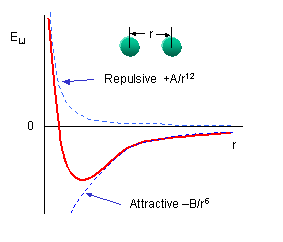# E. Non-Bonded Interaction Energy

$$\newcommand{\vecs}{\overset { \rightharpoonup} {\mathbf{#1}} }$$ $$\newcommand{\vecd}{\overset{-\!-\!\rightharpoonup}{\vphantom{a}\smash {#1}}}$$$$\newcommand{\id}{\mathrm{id}}$$ $$\newcommand{\Span}{\mathrm{span}}$$ $$\newcommand{\kernel}{\mathrm{null}\,}$$ $$\newcommand{\range}{\mathrm{range}\,}$$ $$\newcommand{\RealPart}{\mathrm{Re}}$$ $$\newcommand{\ImaginaryPart}{\mathrm{Im}}$$ $$\newcommand{\Argument}{\mathrm{Arg}}$$ $$\newcommand{\norm}{\| #1 \|}$$ $$\newcommand{\inner}{\langle #1, #2 \rangle}$$ $$\newcommand{\Span}{\mathrm{span}}$$ $$\newcommand{\id}{\mathrm{id}}$$ $$\newcommand{\Span}{\mathrm{span}}$$ $$\newcommand{\kernel}{\mathrm{null}\,}$$ $$\newcommand{\range}{\mathrm{range}\,}$$ $$\newcommand{\RealPart}{\mathrm{Re}}$$ $$\newcommand{\ImaginaryPart}{\mathrm{Im}}$$ $$\newcommand{\Argument}{\mathrm{Arg}}$$ $$\newcommand{\norm}{\| #1 \|}$$ $$\newcommand{\inner}{\langle #1, #2 \rangle}$$ $$\newcommand{\Span}{\mathrm{span}}$$

The non-bonded energy is calculated for all possible pairs of nonbonded atoms, i and j:

Enonbonding = Σi Σj [ -Bij/rij6 +A ij/rij12 ] + Σi Σj (qi qj) / rij

The first term represents van der Waals interactions while the second terms represents coulombic electrostatic interactions.You should remember that van der Waals interactions are short range and occur among all atoms. The 6-12 energy equation based on the Lennard-Jones' potential, shows a negative (attractive) term proportional to -1/r6 and a repulsive term proportional to +1/r12 :I

The A and B parameters control the depth and position (interatomic distance) of the potential energy well for a given pair of non-bonded interacting atoms (e.g. C:C, O:C, etc.). In effect, A determined the degree of stickiness of the van der Waals attraction, and B determines the degree of hardness of the atoms (e.g. marshmallow-like, billiard ball-like, etc.).

The B parameter is related to the "stickiness" of the interactions and is related to the polarization of the atoms. B can be obtained from atomic polarizability measurements, or it can be calculated quantum mechanically. The A parameter is empirically derived to fit nonbonded contacts between atoms in crystal structures.Wolfram Mathematica CDF Player - Interactive Graph of Torsion energy function (free plugin required)

Lennard-Jones Interactive Applet

Coulomb's Law is used to calculate the electrostatic interactions based on appropriate dielectric constants (for buried or water accessible ion-ion pairs).

Enonbonding (electrostatic) = Σi Σj (qi qj) / rij

A higher dielectric constant reflect more shielding by polar solvents of charge pairs. Partial charges on atoms are calculated using ab initio or semiempirical quantum mechanics. (usually MOPAC or AMPAC). The equation for the electrostatic potential is: